# Finding the bisect

To find the bisect of a line you have two choices;

Example 1

Use a protractor.

Find the bisect of the following line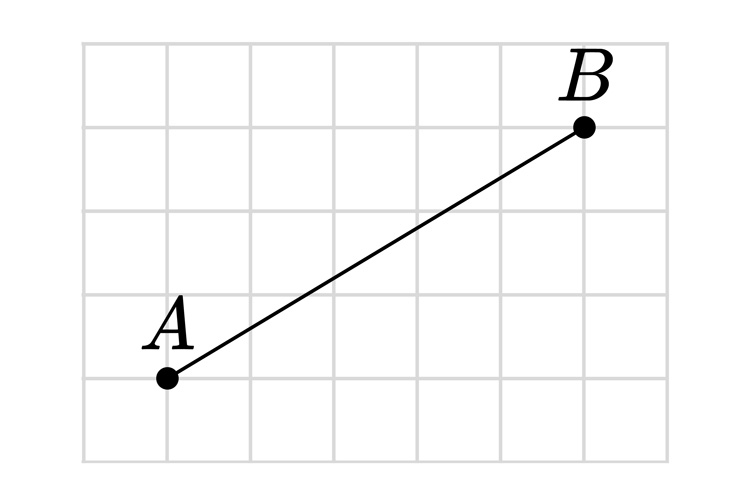Find the half way point between A & B.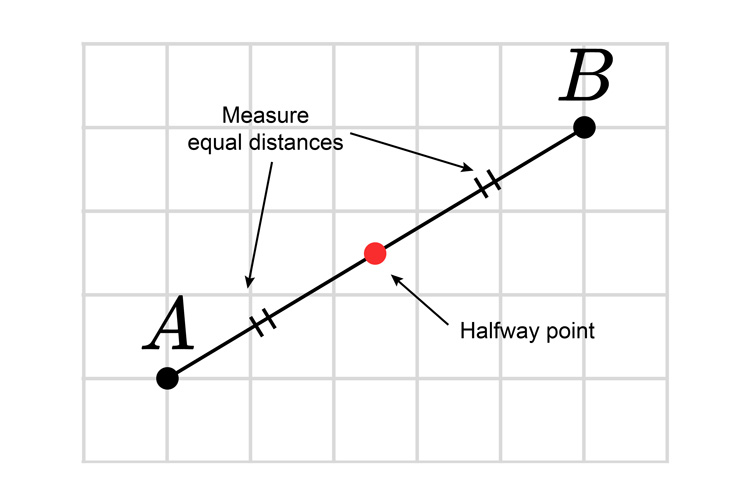Now use a protractor to place a point 90°  to the line A-B.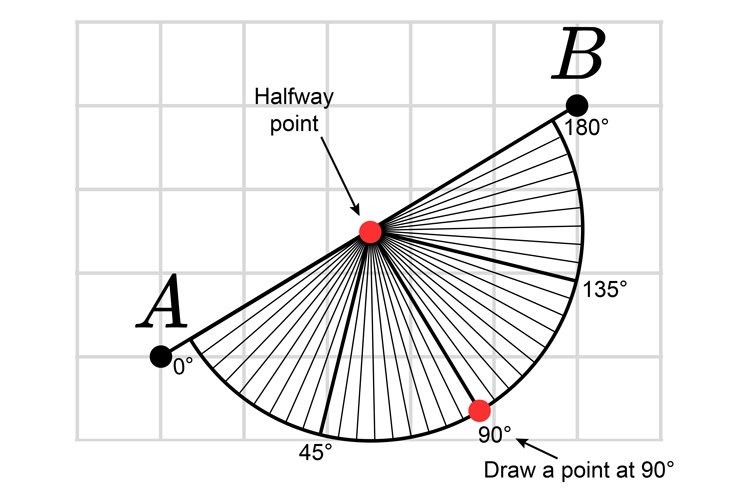Draw a line from the half way point to the 90° point.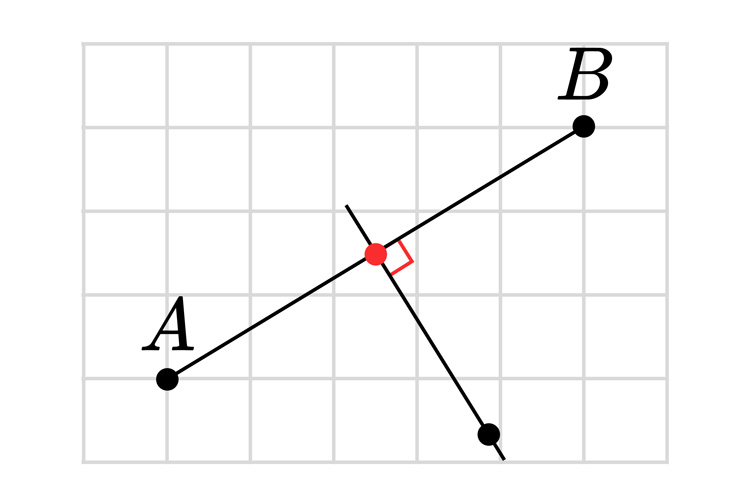This is now a perpendicular bisect of these two points.

Example 2

Use a pencil compass.

Find the bisect of the following line.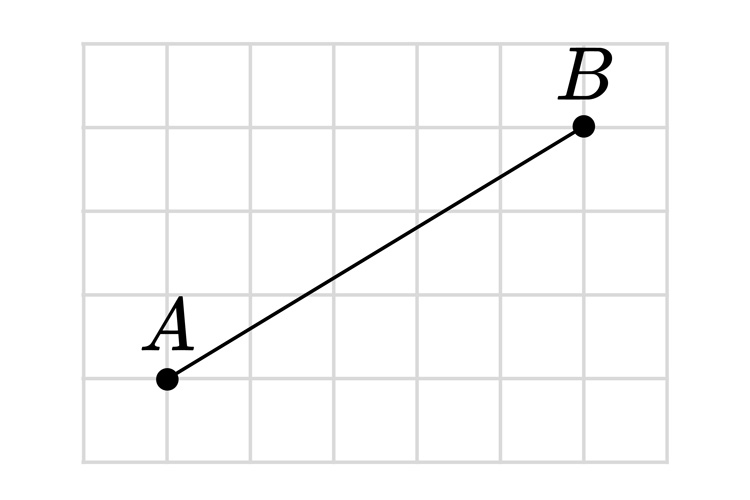Find the half way point between A & B.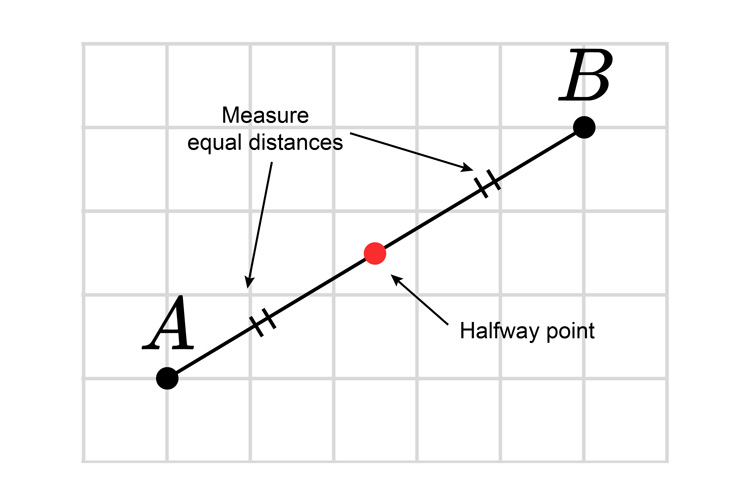Now use a pencil compass that is open more than half way on each point A & B.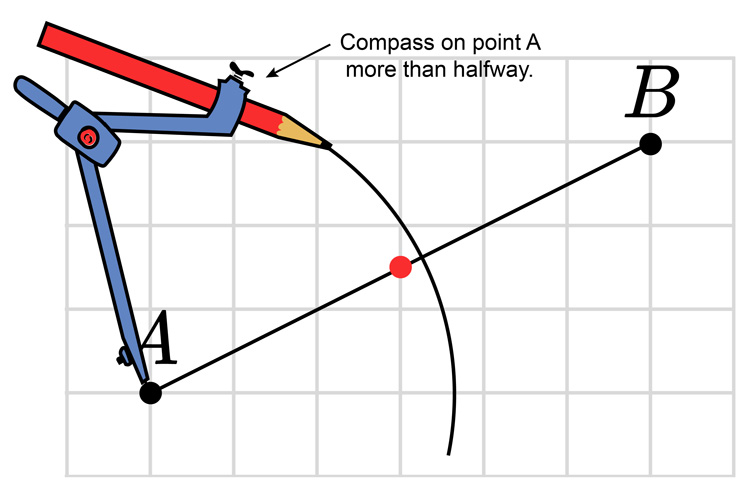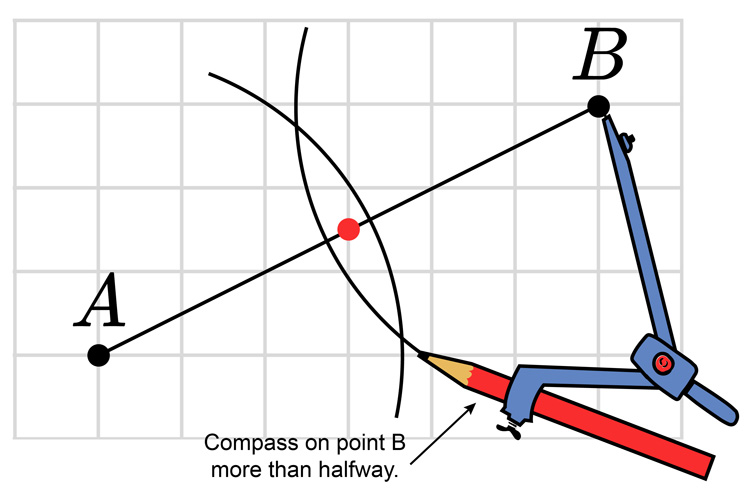Now join the two arc intersections.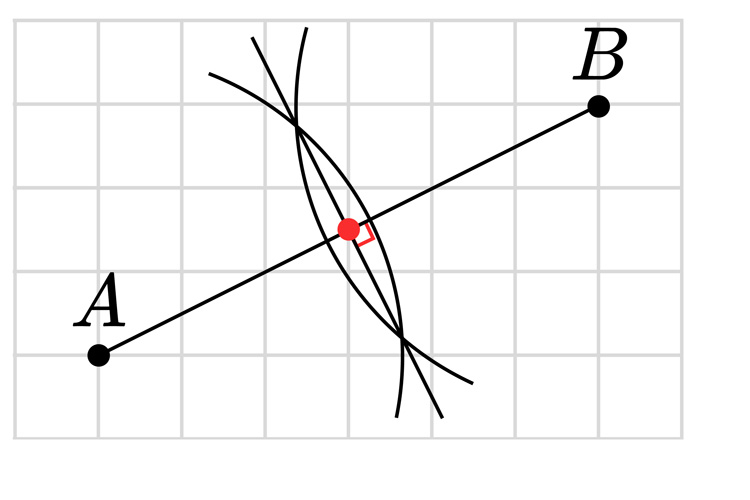This is now the perpendicular bisect of A & B.